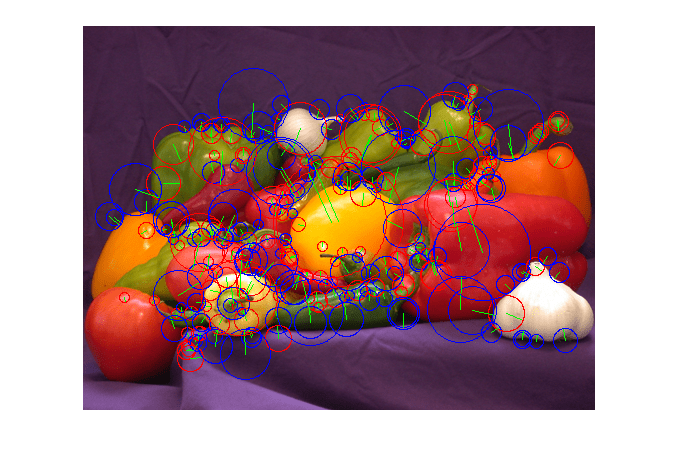# Feature Extraction Using SURF

Object Recognition using Speeded-Up Robust Features (SURF) is composed of three steps: feature extraction, feature description, and feature matching. This example performs feature extraction, which is the first step of the SURF algorithm. The algorithm used here is based on the OpenSURF library implementation. This example shows how you can use GPU Coder™ to solve this compute intensive problem through CUDA® code generation.

### Third-Party Prerequisites

Required

This example generates CUDA MEX and has the following third-party requirements.

• CUDA enabled NVIDIA® GPU and compatible driver.

Optional

For non-MEX builds such as static, dynamic libraries or executables, this example has the following additional requirements.

### Verify GPU Environment

To verify that the compilers and libraries necessary for running this example are set up correctly, use the `coder.checkGpuInstall` function.

```envCfg = coder.gpuEnvConfig('host'); envCfg.BasicCodegen = 1; envCfg.Quiet = 1; coder.checkGpuInstall(envCfg); ```

### Feature Extraction

Feature extraction is a fundamental step in any object recognition algorithm. It refers to the process of extracting useful information referred to as features from an input image. The extracted features must be representative in nature, carrying important and unique attributes of the image.

The SurfDetect.m function is the main entry-point, that performs feature extraction. This function accepts an 8-bit RGB or an 8-bit grayscale image as the input. The output returned is an array of extracted interest points. This function is composed of the following function calls, which contain computations suitable for GPU parallelization:

• The Convert32bitFPGray.m function converts an 8-bit RGB image to an 8-bit grayscale image. If the input provided is already in the 8-bit grayscale format, skip this step. After this step, the 8-bit grayscale image is converted to a 32-bit floating-point representation for enabling fast computations on the GPU.

• The MyIntegralImage.m function calculates the integral image of the 32-bit floating-point grayscale image obtained in the previous step. The integral image is useful for simplifying finding the sum of pixels enclosed within any rectangular region of the image. Finding the sum of pixels helps in improving the speed of convolutions performed in the next step.

• The FastHessian.m function performs convolution of the image with box filters of different sizes and stores the computed responses. For this example, use these parameters:

` Number of Octaves: 5`
` Number of Intervals: 4`
` Threshold: 0.0004`
` Filter Sizes: Octave 1 - 9, 15, 21, 27`
` Octave 2 - 15, 27, 39, 51`
` Octave 3 - 27, 51, 75, 99`
` Octave 4 - 51, 99, 147, 195`
` Octave 5 - 99, 195, 291, 387`
• The NonMaxSuppression_gpu.m function performs non-maximal suppression to filter out only the useful interest points from the responses obtained earlier. To generate a kernel that uses the `atomicAdd` operation, use the `coder.ceval` construct. Because this construct is not compatible when invoked directly from MATLAB®, there are two different function calls. The NonMaxSuppression_gpu.m function is invoked when GPU code generation is enabled and the NonMaxSuppression.m is invoked when you are executing the algorithm directly in MATLAB.

• The OrientationCalc.m function calculates and assigns orientation to the interest points in the previous step.

The final result is an array of interest points where an interest point is a structure that consists of these fields:

` x, y (coordinates), scale, orientation, Laplacian`

Read an input image into MATLAB by using the `imread` function.

```imageFile = 'peppers.png'; inputImage = imread(imageFile); imshow(inputImage); ```### Generate CUDA MEX for the Function

To generate CUDA MEX for the `SurfDetect` function, create a GPU Coder configuration object, and then run the `codegen` function.

```cfg = coder.gpuConfig('mex'); evalc('codegen -config cfg SurfDetect -args {inputImage}'); ```

### Run the MEX Function on a GPU

You can invoke the generated MEX function `SurfDetect_mex` to run on a GPU:

```disp('Running GPU Coder SURF'); interestPointsGPU = SurfDetect_mex(inputImage); fprintf(' GPU Coder SURF found: %d interest points\n',length(interestPointsGPU)); ```
```Running GPU Coder SURF GPU Coder SURF found: 249 interest points ```

### Depict the Extracted Interest Points

The output `interestPointsGPU` is an array of extracted interest points. These interest points are depicted over the input image in a figure window.

```DrawIpoints(imageFile, interestPointsGPU); ```### References

1. Notes on the OpenSURF Library by Christopher Evans.

2. SURF: Speeded-Up Robust Features by Herbert Bay, Tinne Tuytelaars, and Luc Van Gool.